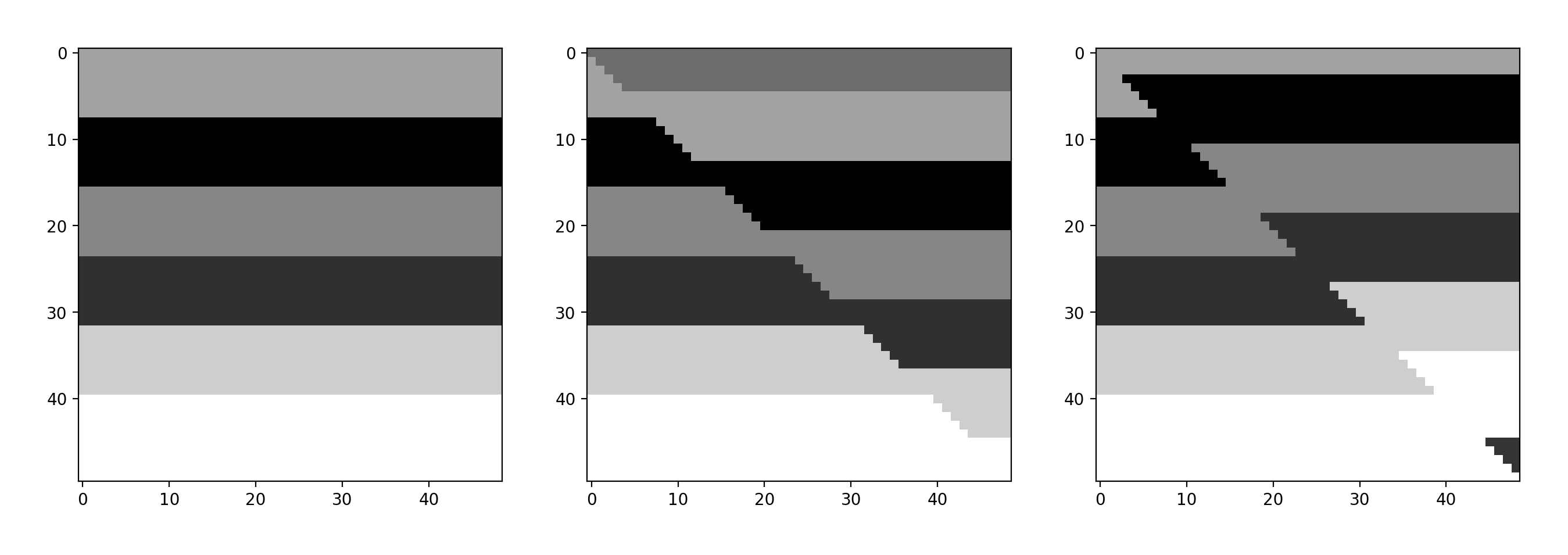# Adding normal and reverse faults to an impedance model with Python

This is a short and sweet follow-up to yesterday’s post Making many synthetic seismic models in Python, in which I want to show how to add oblique faults to an impedance model, as opposed to a vertical one.

In the pseudo-code below, with the first line of code I select one of the impedance models from the many I created, then in lines 2 and 3, respectively, make two new versions, one shifted up 5 samples, one shifted down 5 samples. The impedance values for the extra volume added – at the bottom in the first case, the top in the second – are derived from the unshifted impedance model.

Next, I make two copies of the original, call them normal and reverse, and then replace the upper triangle with the upper triangle of the shifted down and shifted up versions, respectively.

```unshifted = impedances[n]
up = sp.ndimage.interpolation.shift(unshifted, (5,0), cval=unshifted[0,0] * 0.9)
down = sp.ndimage.interpolation.shift(unshifted, (-5,0), cval=unshifted[0,-1] * 0.8)
normal = copy.deepcopy(unshifted)
reverse = copy.deepcopy(unshifted)
sz = len(unshifted)-1
normal[np.triu_indices(sz)] = up[np.triu_indices(sz)]
reverse[np.triu_indices(sz)] = down[np.triu_indices(sz)]```

Done!
The results are shown in the figure below.Left: unfaulted impedance model; center, model with normal fault; right, model with reverse fault.

## 2 thoughts on “Adding normal and reverse faults to an impedance model with Python”

1.agileevan on said:

(np.triu_indicies)[https://docs.scipy.org/doc/numpy-1.10.4/reference/generated/numpy.triu_indices.html], I learned something new?

Next, how about using np.argwhere() to return the indicies above or below a curved listric fault, or antithetic fault…I can almost see it now!

•matteomycarta on said:

I like the idea of `np.argwhere()` Evan… I was also thinking of thrust faults with ramp-flat-ramp geometry, all these are very doable. Perhaps, though, as Matt has commented on the SU slack channel, probably at some point might as well be working in `pynoddy`….